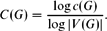Home

# On the Normalized Shannon Capacity of a Union

## Abstract

Let G 1 × G 2 denote the strong product of graphs G 1 and G 2, that is, the graph on V(G 1) × V(G 2) in which (u 1, u 2) and (v 1, v 2) are adjacent if for each i = 1, 2 we have ui = vi or u i v iE(G i ). The Shannon capacity of G is c(G) = limn → ∞ α(Gn )1/n , where Gn denotes the n-fold strong power of G, and α(H) denotes the independence number of a graph H. The normalized Shannon capacity of G is$$C(G) = \ffrac {\log c(G)}{\log |V(G)|}.$$
Alon  asked whether for every ε < 0 there are graphs G and G′ satisfying C(G), C(G′) < ε but with C(G + G′) > 1 − ε. We show that the answer is no.

Hide All

# On the Normalized Shannon Capacity of a Union

## Metrics

### Full text viewsFull text views reflects the number of PDF downloads, PDFs sent to Google Drive, Dropbox and Kindle and HTML full text views.

Total number of HTML views: 0
Total number of PDF views: 0 *Loading metrics...

### Abstract viewsAbstract views reflect the number of visits to the article landing page.

Total abstract views: 0 *Loading metrics...

* Views captured on Cambridge Core between <date>. This data will be updated every 24 hours.

Usage data cannot currently be displayed.